A black body is one that absorbs all the EM radiation (light...) that strikes it. To stay in thermal equilibrium, it must emit radiation at the same rate as it absorbs it so a black body also radiates well. (Stoves are black.)

Radiation from a hot object is familiar to us. Objects around room temperature radiate mainly in the infrared as seen the the graph below.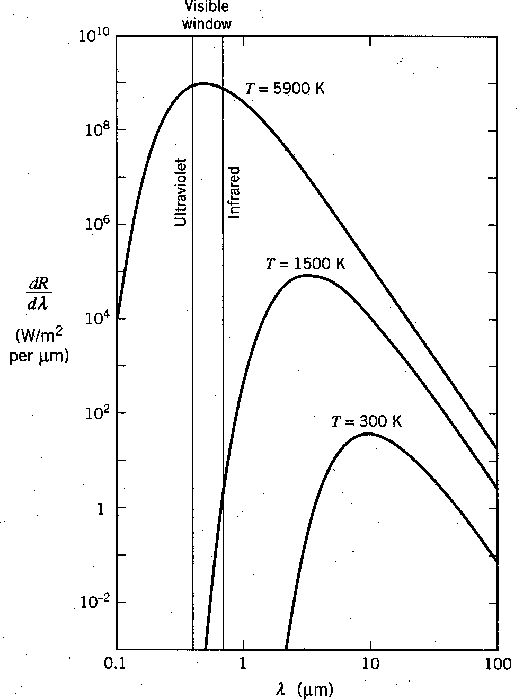If we heat an object up to about 1500 degrees we will begin to see a dull red glow and we say the object is red hot. If we heat something up to about 5000 degrees, near the temperature of the sun's surface, it radiates well throughout the visible spectrum and we say it is white hot.

By considering plates in thermal equilibrium it can be shown that the emissive power over the absorption coefficient must be the same as a function of wavelength, even for plates of different materials.It there were differences, there could be a net energy flow from one plate to the other, violating the equilibrium condition.A black body is one that absorbs all radiation incident upon it.Thus, the black body Emissive power,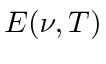, is universal and can be derived from first principles.

A good example of a black body is a cavity with a small hole in it. Any light incident upon the hole goes into the cavity and is essentially never reflected out since it would have to undergo a very large number of reflections off walls of the cavity. If we make the walls absorptive (perhaps by painting them black), the cavity makes a perfect black body.There is a simple relation between the energy density in a cavity,, and the black body emissive power of a black body which simply comes from an analysis of how much radiation, traveling at the speed of light, will flow out of a hole in the cavity in one second.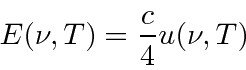The only part that takes a little thinking is the 4 in the equation above.

Rayleigh and Jeans calculated t he energy density (in EM waves) inside a cavity and hence the emission spectrum of a black body. Their calculation was based on simple EM theory and equipartition. It not only did not agree with data; it said that all energy would be instantly radiated away in high frequency EM radiation. This was called the ultraviolet catastrophe.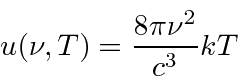Plank found a formula that fit the data well at both long and short wavelength.His formula fit the data so well that he tried to find a way to derive it. In a few months he was able to do this, by postulating that energy was emitted in quanta with. Even though there are a very large number of cavity modes at high frequency, the probability to emit such high energy quanta vanishes exponentially according to the Boltzmann distribution. Plank thus suppressed high frequency radiation in the calculation and brought it into agreement with experiment. Note that Plank's Black Body formula is the same in the limit that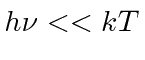but goes to zero at large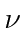while the Rayleigh formula goes to infinity.It is interesting to note that classical EM waves would suck all the thermal energy out of matter, making the universe a very cold place for us. The figure below compares the two calculations to some data at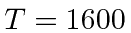degrees. (It is also surprising that the start of the Quantum revolution came from Black Body radiation.)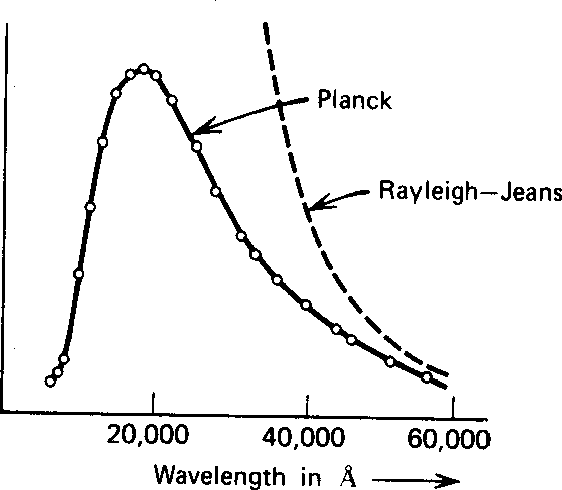So the emissive power per unit area isWe can integrate this over frequency to get the total power radiated per unit area.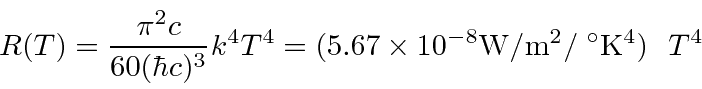* Example: What is the temperature at the solar surface? Use both the the intensity of radiation on earth and that the spectrum peaks about 500 nm to get answers.*

* Example: The cosmic microwave background is black body radiation with a temperature of 2.7 degrees. For what frequency (and what wavelength) does the intensity peak?*

Jim Branson 2013-04-22